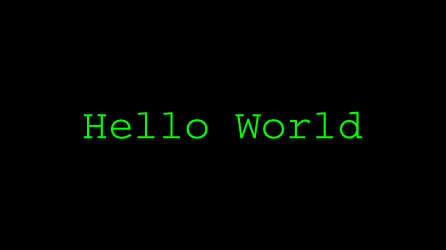## [SICP 1.2.4] Exercise 1.17 Memo

5-12-2014

Exercise 1.17. Using the results of exercises 1.16 and 1.17, devise a procedure that generates an iterative process for multiplying two integers in terms of adding, doubling, and halving and uses a logarithmic number of steps.

In the exercise, the multiplication is implemented as follows:

```(define (mul-1 a b)
(if (= b 0) 0 (+ a (mul-1 a (- b 1))))
)
```

This requires the order of b. The question here is that we want to implement the same function that requires the order proportional to some logarithm.

```(define (double x) (+ x x))
(define (halve x) (/ x 2))
(define (mul-2 a b)
(cond ((= b 0) 0)
((even? b) (mul-2 (double a) (halve b)))
(else (+ a (mul-2 a (- b 1)))))
)
```

This only requires the order of log_2(b).

As a result, we get the following output:

```(mul-1 100 5000000) ; memory shortage
(mul-2 100 5000000) ; 500000000
(mul-2 10000 50000000000000) ; 500000000000000000
```

## Time vs Space

There are two important resources according to [SICP - 1.2 Procedures and the Processes They Generate ] – that is,

Time: Time to execute some procedure . As steps grows, so does the time, following an order like n, n^2, log n and so on.

Space: Space to use. Then what’s “the Space”? It seems to me that it’s like memory space in an computer ( Sorry if my understanding is wrong ). The memory region is provided for each computer processes by the operating system, and each program runs in its given range. When the spaces required by a program exceeds that capacity, the memory leak occurs.

These two resources have a key for understanding the difference between linear recursive process and linear iterative process.

## Linear Recursive Process vs Linear Iterative Process

To disclose actual difference between these two processes, let’s consider the following code.

In terms of the time, both processes increase their value proportional to n. However, you can see the complete difference in the space.

```
; Linear recursive process
; from the figure 1.3 in SICP

(factorial 6)
(* 6 (factorial 5))
(* 6 (* 5 (factorial 4)))
(* 6 (* 5 (* 4 (factorial 3))))
(* 6 (* 5 (* 4 (* 3 (factorial 2)))))
(* 6 (* 5 (* 4 (* 3 (* 2 (factorial 1))))))
(* 6 (* 5 (* 4 (* 3 (* 2 1)))))
(* 6 (* 5 (* 4 (* 3 2))))
(* 6 (* 5 (* 4 6)))
(* 6 (* 5 24))
(* 6 120)
720

```

The linear recursive process increases the space for operation proportional to n, while the space of linear iterative process doesn’t.

```
; Linear iterative process
; from the figure 1.4 in SICP

(factorial 6)
(fact-iter 1 1 6)
(fact-iter 1 2 6)
(fact-iter 2 3 6)
(fact-iter 6 4 6)
(fact-iter 24 5 6)
(fact-iter 120 6 6)
(fact-iter 720 7 6)
720

```

## First Appearance of This Blog

5-9-2014## What is this blog about?

I’m a second year web engineer who graduated a university in Japan.

My major wasn’t science, and I hadn’t taken much lectures about science.

After graduation, I began to feel that even if the technologies around us change its forms, the essential part of it doesn’t change, like computers has changed its forms and improved its performance over and over again in previous decades but C, moreover, Assembly still remain as the infrastructure of that technologies.

So what is the essential part of the technologies?  I think we can’t talk about this without theoretical parts of computer science, or even can’t hatch the technologies in next generation. I know some people say that theory without practice won’t make it, but I think I can say the same thing for practice without theory. While agile development is in a main stream and people became able to release smaller web services experimentally, its background of specifications should be based on some hypotheses with theories.

So I’ve decided to write a blog for organizing what I’ll study about computer science, some mathematics and physics.